xScaling (geometry)Encyclopedia
In Euclidean geometry
Euclidean geometry
Euclidean geometry is a mathematical system attributed to the Alexandrian Greek mathematician Euclid, which he described in his textbook on geometry: the Elements. Euclid's method consists in assuming a small set of intuitively appealing axioms, and deducing many other propositions from these...

, uniform scaling (isotropic scaling, homogeneous dilation, homothety) is a linear transformation
Linear transformation
In mathematics, a linear map, linear mapping, linear transformation, or linear operator is a function between two vector spaces that preserves the operations of vector addition and scalar multiplication. As a result, it always maps straight lines to straight lines or 0...

that enlarges (increases) or shrinks (diminishes) objects by a scale factor
Scale factor
A scale factor is a number which scales, or multiplies, some quantity. In the equation y=Cx, C is the scale factor for x. C is also the coefficient of x, and may be called the constant of proportionality of y to x...

that is the same in all directions. The result of uniform scaling is similar
Similarity (geometry)
Two geometrical objects are called similar if they both have the same shape. More precisely, either one is congruent to the result of a uniform scaling of the other...

(in the geometric sense) to the original. A scale factor of 1 is normally allowed, so that congruent shapes are also classed as similar. (Some school text books specifically exclude this possibility, just as some exclude squares from being rectangles or circles from being ellipses.)

More general is scaling with a separate scale factor for each axis direction. Non-uniform scaling (anisotropic scaling, inhomogeneous dilation) is obtained when at least one of the scaling factors is different from the others; a special case is directional scaling or stretching (in one direction). Non-uniform scaling changes the shape
Shape
The shape of an object located in some space is a geometrical description of the part of that space occupied by the object, as determined by its external boundary – abstracting from location and orientation in space, size, and other properties such as colour, content, and material...

of the object; e.g. a square may change into a rectangle, or into a parallelogram if the sides of the square are not parallel to the scaling axes (the angles between lines parallel to the axes are preserved, but not all angles).

## Matrix representation

A scaling can be represented by a scaling matrix. To scale an object by a vector v = (vx, vy, vz), each point p = (px, py, pz) would need to be multiplied with this scaling matrix: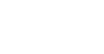As shown below, the multiplication will give the expected result: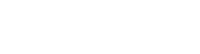Such a scaling changes the diameter
Diameter
In geometry, a diameter of a circle is any straight line segment that passes through the center of the circle and whose endpoints are on the circle. The diameters are the longest chords of the circle...

of an object by a factor between the scale factors, the area
Area
Area is a quantity that expresses the extent of a two-dimensional surface or shape in the plane. Area can be understood as the amount of material with a given thickness that would be necessary to fashion a model of the shape, or the amount of paint necessary to cover the surface with a single coat...

by a factor between the smallest and the largest product of two scale factors, and the volume
Volume
Volume is the quantity of three-dimensional space enclosed by some closed boundary, for example, the space that a substance or shape occupies or contains....

by the product of all three.

The scaling is uniform if and only if
If and only if
In logic and related fields such as mathematics and philosophy, if and only if is a biconditional logical connective between statements....

the scaling factors are equal (vx = vy = vz). If all except one of the scale factors are equal to 1, we have directional scaling.

In the case where vx = vy = vz = k, the scaling is also called an enlargement or dilation by a factor k, increasing the area by a factor of k2 and the volume by a factor of k3.

A scaling in the most general sense is any affine transformation
Affine transformation
In geometry, an affine transformation or affine map or an affinity is a transformation which preserves straight lines. It is the most general class of transformations with this property...

with a diagonalizable matrix
Diagonalizable matrix
In linear algebra, a square matrix A is called diagonalizable if it is similar to a diagonal matrix, i.e., if there exists an invertible matrix P such that P −1AP is a diagonal matrix...

. It includes the case that the three directions of scaling are not perpendicular. It includes also the case that one or more scale factors are equal to zero (projection
Projection (linear algebra)
In linear algebra and functional analysis, a projection is a linear transformation P from a vector space to itself such that P2 = P. It leaves its image unchanged....

), and the case of one or more negative scale factors. The latter corresponds to a combination of scaling proper and a kind of reflection: along lines in a particular direction we take the reflection in the point of intersection with a plane that need not be perpendicular; therefore it is more general than ordinary reflection in the plane.

## Using homogeneous coordinates

In projective geometry
Projective geometry
In mathematics, projective geometry is the study of geometric properties that are invariant under projective transformations. This means that, compared to elementary geometry, projective geometry has a different setting, projective space, and a selective set of basic geometric concepts...

, often used in computer graphics
Computer graphics
Computer graphics are graphics created using computers and, more generally, the representation and manipulation of image data by a computer with help from specialized software and hardware....

, points are represented using homogeneous coordinates
Homogeneous coordinates
In mathematics, homogeneous coordinates, introduced by August Ferdinand Möbius in his 1827 work Der barycentrische Calcül, are a system of coordinates used in projective geometry much as Cartesian coordinates are used in Euclidean geometry. They have the advantage that the coordinates of points,...

. To scale an object by a vector v = (vx, vy, vz), each homogeneous coordinate vector p = (px, py, pz, 1) would need to be multiplied with this projective transformation matrix: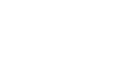As shown below, the multiplication will give the expected result: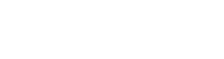Since the last component of a homogeneous coordinate can be viewed as the denominator of the other three components, a uniform scaling by a common factor s (uniform scaling) can be accomplished by using this scaling matrix: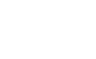For each vector p = (px, py, pz, 1) we would havewhich would be homogenized to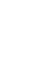• Scale (ratio)
Scale (ratio)
The scale ratio of some sort of model which represents an original proportionally is the ratio of a linear dimension of the model to the same dimension of the original. Examples include a 3-dimensional scale model of a building or the scale drawings of the elevations or plans of a building. In such...

• Scale (map)
Scale (map)
The scale of a map is defined as the ratio of a distance on the map to the corresponding distance on the ground.If the region of the map is small enough for the curvature of the Earth to be neglected, then the scale may be taken as a constant ratio over the whole map....

• Scales of scale models
• Scale (disambiguation)
• Scaling in gravity
• Transformation matrix